+92(42)37241334-5

## Details of Prize Bonds

Prize Bonds of Pakistan are very famous and considered to be a good and safe investment. It is secured investment because the Government of Pakistan bearer security and responsibility. Prize Bonds are available in the worth of RS 100, 200, 750, 1500, 2500, 15000 and prime bonds of 25000 and 40,000 respectively.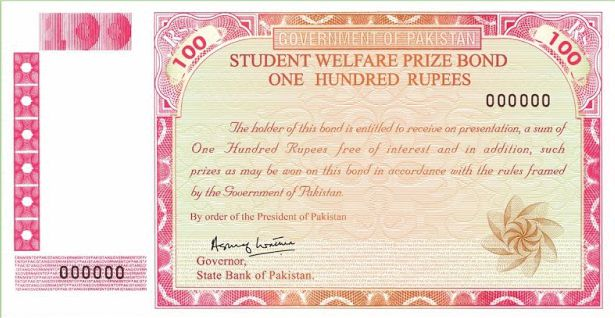Bond Value: Rs. 100 (Student Welfare Prize Bond) Prize Position Total Prizes Prize Value First Prize 01 Rs. 700,000 Second Prize 03 Rs. 200,000 Third Prize 1199 Rs. 1000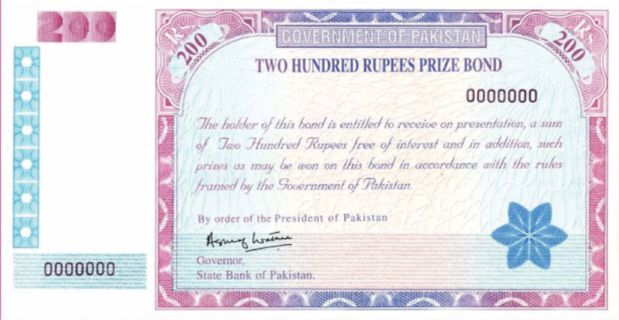Bond Value: Rs. 200 (Two Hundred Rupees Prize Bond) Prize Position Total Prizes Prize Value First Prize 01 Rs. 750,000 Second Prize 05 Rs. 250,000 Third Prize 2394 Rs. 1250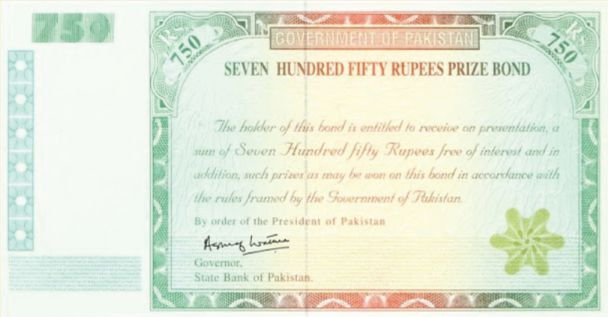Bond Value: Rs. 750 (Seven Hundred Fifty Rupees Prize Bond) Prize Position Total Prizes Prize Value First Prize 01 Rs. 1500,000 Second Prize 03 Rs. 500,000 Third Prize 1696 Rs. 9300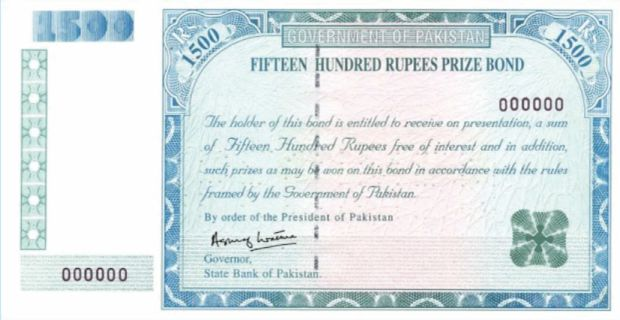Bond Value: Rs. 1500 (Fifteen Hundred Rupees Prize Bond) Prize Position Total Prizes Prize Value First Prize 01 Rs. 30,00,000 Second Prize 03 Rs. 10,00,000 Third Prize 1696 Rs. 18500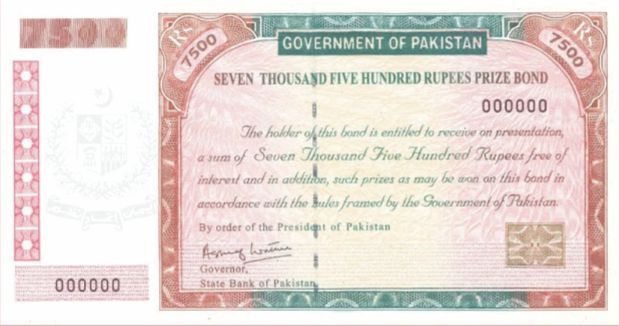Bond Value: Rs. 7500 (Seven Thousand Five Hundred Rupees Prize Bond) Prize Position Total Prizes Prize Value First Prize 01 Rs. 150,00,000 Second Prize 03 Rs. 50,00,000 Third Prize 1696 Rs. 93000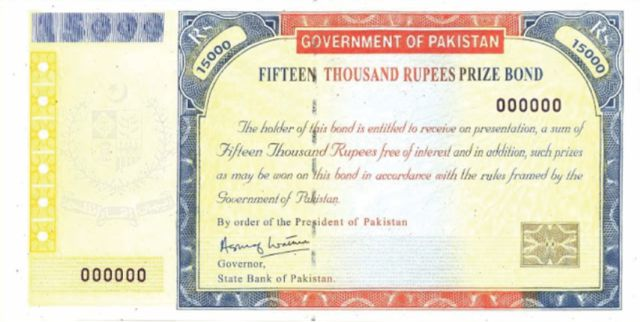Bond Value: Rs. 15000 (Fifteen Thousand Rupees Prize Bond) Prize Position Total Prizes Prize Value First Prize 01 Rs. 30,000,000 Second Prize 03 Rs. 10,000,000 Third Prize 1696 Rs. 185000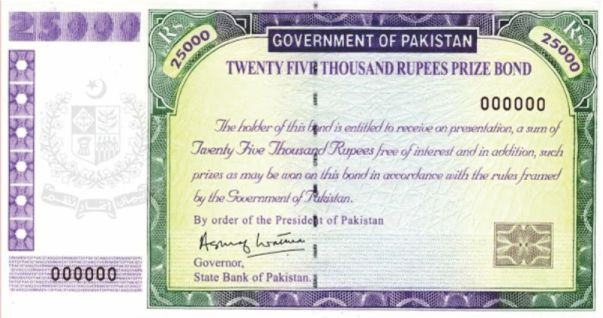Bond Value: Prime Rs. 25000 (Twenty Five Thousand Rupees Prize Bond) Prize Position Total Prizes Prize Value First Prize 01 Rs. 50,000,000 Second Prize 03 Rs. 15,000,000 Third Prize 1696 Rs. 3,12,000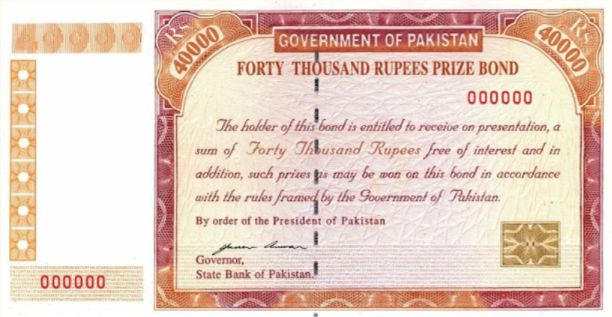Bond Value: Prime Rs. 40000 (Twenty Five Thousand Rupees Prize Bond) Prize Position Total Prizes Prize Value First Prize 01 Rs. 75,000,000 Second Prize 03 Rs. 25,000,000 Third Prize 1696 Rs. 500,000